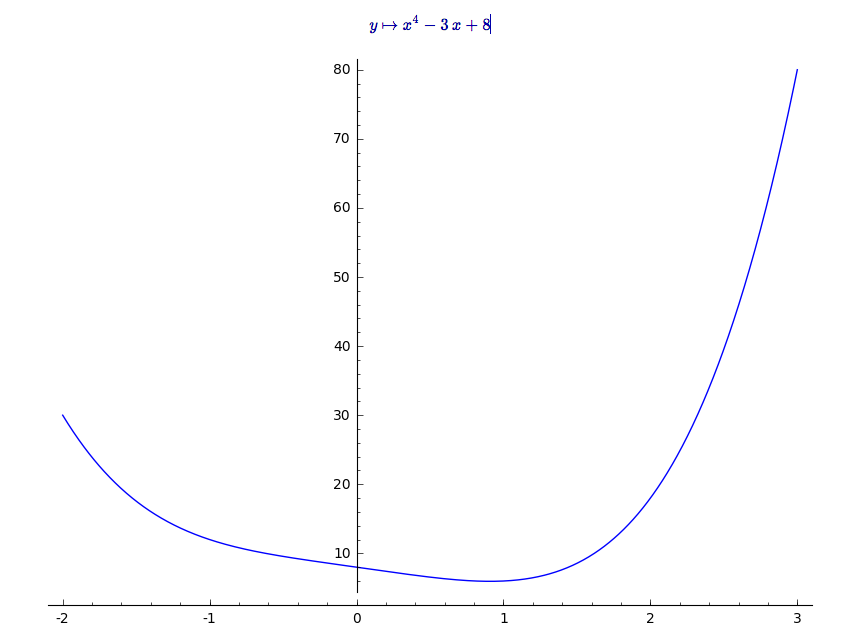# Revision history [back]

Would this give you what you are looking for? (assuming your interval is [-1;2]

f(y) = x^4-3*x+8
f.show()
find_local_maximum(f, -1, 2)


I am generally big on plotting unction like this, you can try to get a feel of your function

plot(f(y), -2,3)I highly recommend the SageMath Calculus Tutorial if you at all have the time.

Would this give you what you are looking for? (assuming your interval is [-1;2]

f(y) = x^4-3*x+8
f.show()
find_local_maximum(f, -1, 2)


I am generally big on plotting unction like this, you can try to get a feel of your function

plot(f(y), -2,3)I highly recommend the SageMath Calculus Tutorial if you at all have the time.

### Update,

f = (1/4)*log(2.02000000000000*x + 1) + (1/2)*log(0.00999999999999979*x + 1) + (1/4)*log(-2*x + 1)

(95160969.23167175, 0.50000000262712752)